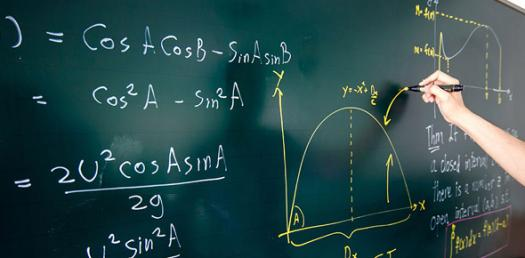# Algebra 1 Practice Quiz

15 Questions | Total Attempts: 1435SettingsCreate your own QuizThis is a quiz covering topics from the first semester of Algebra 1.

• 1.
The ordered pair (3,0) is found:
• A.

On the y-axis

• B.

In the first Quadrant

• C.

In the fourth Quadrant

• D.

On the x-axis

• 2.
(-3, 7) is located in quadrant:
• A.

I

• B.

II

• C.

III

• D.

IV

• 3.
The price of a house increased by 7%. If the new price is \$107,000, what was the original cost of the house?
• A.

\$100,000

• B.

\$114,490

• C.

\$99,510

• D.

\$93,000

• 4.
(8,2) is a solution of x - 3y = 6
• A.

True

• B.

False

• 5.
The graph of y = 2x + 3 passes through the origin.
• A.

True

• B.

False

• 6.
What is the y- intercept for 5y = 4x - 20?
• A.

(5,0)

• B.

(0,5)

• C.

(-4,0)

• D.

(0,-4)

• 7.
What quadrant does the line 5x + 3y = 15 not pass through?
• A.

I

• B.

II

• C.

III

• D.

IV

• 8.
What is the x-intercept of x =2?
• A.

(0,2)

• B.

(2,0)

• C.

(0,0)

• D.

There is no x-intercept

• 9.
What is the slope of the line through (6,5) and (9,1)?
• A.

4/3

• B.

-3/4

• C.

-4/3

• D.

3/4

• 10.
What is the slope of the line x = 7?
• A.

7

• B.

0

• C.

1/7

• D.

Undefined

• 11.
Find the slope of the line 6x-2y=12-2y?
• A.

6

• B.

1/6

• C.

0

• D.

Undefined

• 12.
Find an equation for the line with slope and y-intercept 3.
• A.

4x - 3y = -3

• B.

4x - 3y = -9

• C.

3y - 4x = -3

• D.

3y - 4x = -9

• 13.
Find an equation of the line through (25) with a slope of .
• A.

Y - x = 4

• B.

2y - 3x = 4

• C.

3x - 2y = 4

• D.

X - y = 4

• 14.
Find an equation of a line through (3,4) and (1,-7).
• A.

11x - 2y = -5

• B.

11x - 2y = -1

• C.

2x - 11y = -38

• D.

11x - 2y = 25

• 15.
Find an equation of a line through (0,2) parallel to the line 3x-6y=1.
• A.

2y - x = 4

• B.

2y x = 2x

• C.

Y - 2x = 2

• D.

Y - 2x = 4

Related TopicsBack to top
×

Wait!
Here's an interesting quiz for you.